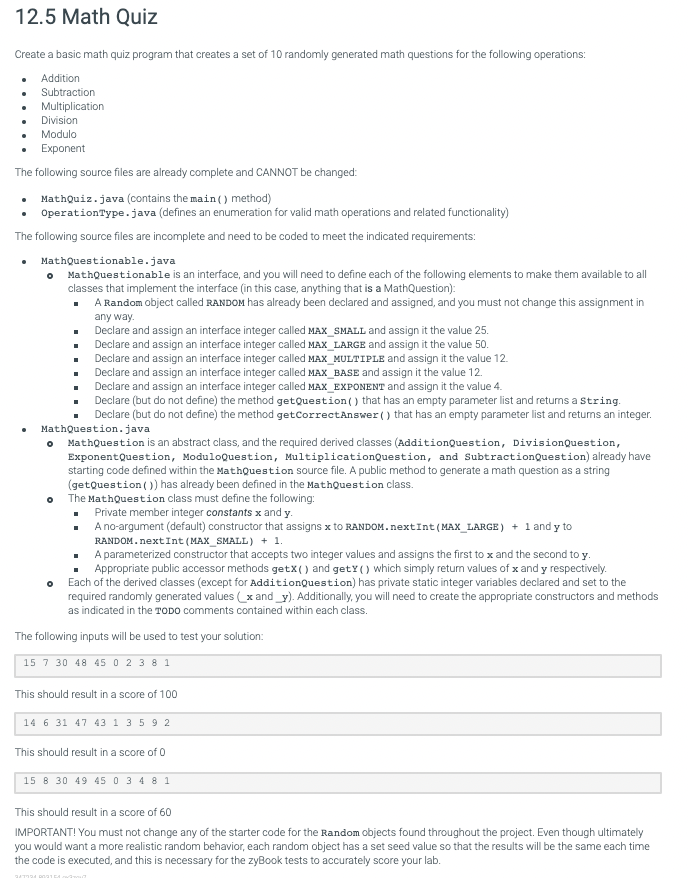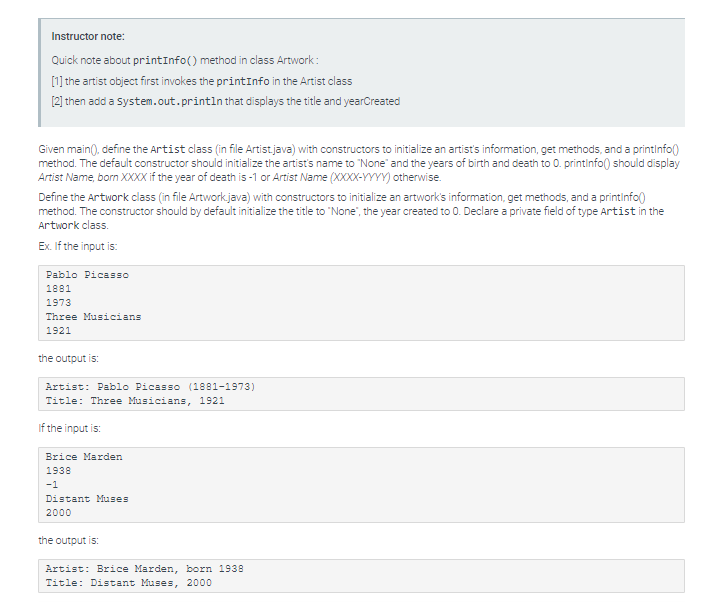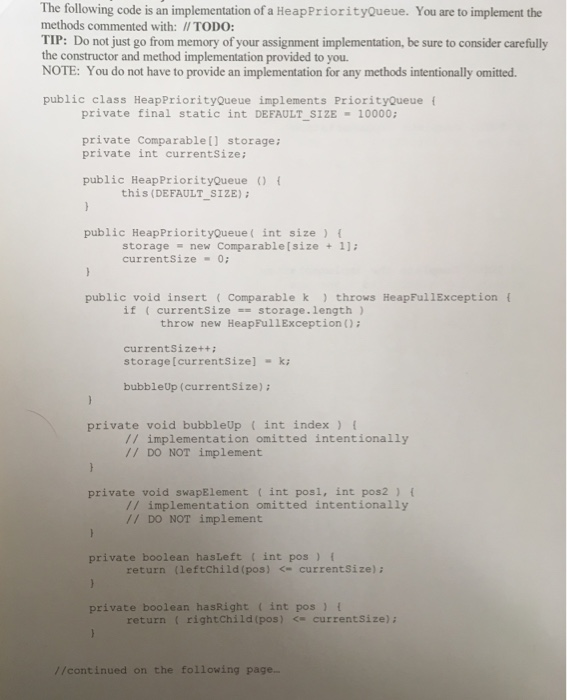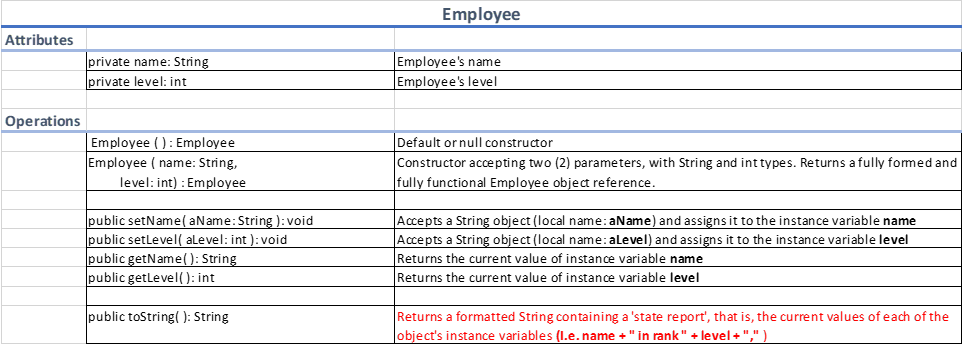# Java Programming: Math QuizStarting codes:

MathQuiz.java

import java.util.Scanner;

public class MathQuiz {

private final int NUMBER_OF_QUESTIONS = 10;

private final MathQuestion[] questions = new MathQuestion[NUMBER_OF_QUESTIONS];

private final int[] userAnswers = new int[NUMBER_OF_QUESTIONS];

public static void main(String[] args) {

MathQuiz app = new MathQuiz();

System.out.println(app.welcomeAndInstructions());

app.createQuiz();

}

private String welcomeAndInstructions() {

return "Welcome to Math Quiz!\n" +

"There are currently six types of math questions possible:\n" +

"Addition, Subtraction, Multiplication, Exponents, Division, and Modulo.\n" +

"The answer to each question will always be an integer.\n\n" +

"Good luck!\n";

}

private void createQuiz() {

int i = 0;

OperationType op;

while (i < NUMBER_OF_QUESTIONS) {

op = OperationType.getRandom();

switch (op) {

break;

case SUBTRACT:

questions[i++] = new SubtractionQuestion();

break;

case MULTIPLY:

questions[i++] = new MultiplicationQuestion();

break;

case EXPONENT:

questions[i++] = new ExponentQuestion();

break;

case DIVIDE:

questions[i++] = new DivisionQuestion();

break;

case MODULO:

questions[i++] = new ModuloQuestion();

break;

default:

throw new IllegalArgumentException();

}

}

}

Scanner scanner = new Scanner(System.in);

int questionNum = 1;

for (MathQuestion q : questions) { //POLYMORPHISM!!

System.out.printf("Question %2d:  %s ", questionNum, q.getQuestion());

questionNum++;

}

}

int numberCorrect = 0;

String question;

for (int i = 0; i < NUMBER_OF_QUESTIONS; i++) {

question = questions[i].getQuestion();

System.out.printf("Question number %d:\n    %s\n", i + 1, question);

System.out.println("    You were CORRECT.");

numberCorrect++;

} else {

System.out.printf("    You said %d, which is INCORRECT.\n", userAnswers[i]);

}

}

System.out.printf("\nYou got %d questions correct.\n", numberCorrect);

}

}

MathQuestion.java

public abstract class MathQuestion implements MathQuestionable {

// TODO: Declare private member integer constants x and y

// TODO: Define a default (no parameters) constructor that assigns

// x to RANDOM.nextInt(MAX_LARGE) + 1

// y to RANDOM.nextInt(MAX_SMALL) + 1

// TODO: Define a parameterized constructor that accepts two integer values

// The first parameter must be assigned to x

// The second parameter must be assigned to y

// TODO: Define public accessor methods getX() and getY()

public String getQuestion(OperationType op) {

return "What is " + x + ' ' + op.getSymbol() + ' ' + y + '?';

}

}

// A video walk-through will be provided for how to write the code for this class

}

class DivisionQuestion extends MathQuestion {

private static int _x = RANDOM.nextInt(MAX_LARGE) + 1;

private static int _y = RANDOM.nextInt(_x) + 1;

// TODO: Define default constructor to call parent class constructor and passing _x and _y as arguments

// You will also need to reassign new random values to both _x and _y as shown in the initial assignment statements

// TODO: Define a parameterized constructor that accepts two integers and passes these in a call to the parent constructor

// TODO: Define getQuestion that returns a String and makes a call to the parent getQuestion method.

// You will need to pass OperationType.DIVIDE as the argument

// TODO: Define getCorrectAnswer that returns an integer result of x / y (this is not as simple as it looks!)

}

class ExponentQuestion extends MathQuestion {

private static int _x = RANDOM.nextInt(MAX_BASE) + 1;

private static int _y = RANDOM.nextInt(MAX_EXPONENT);

// TODO: Define default constructor to call parent class constructor and passing _x and _y as arguments

// You will also need to reassign new random values to both _x and _y as shown in the initial assignment statements

// TODO: Define a parameterized constructor that accepts two integers and passes these in a call to the parent constructor

// TODO: Define getQuestion that returns a String and makes a call to the parent getQuestion method.

// You will need to pass OperationType.EXPONENT as the argument

// TODO: Define getCorrectAnswer that returns an integer result of Math.pow(x, y) (this is not as simple as it looks!)

}

class ModuloQuestion extends MathQuestion {

private static int _x = RANDOM.nextInt(MAX_LARGE) + 1;

private static int _y = RANDOM.nextInt(_x) + 1;

// TODO: Define default constructor to call parent class constructor and passing _x and _y as arguments

// You will also need to reassign new random values to both _x and _y as shown in the initial assignment statements

// TODO: Define a parameterized constructor that accepts two integers and passes these in a call to the parent constructor

// TODO: Define getQuestion that returns a String and makes a call to the parent getQuestion method.

// You will need to pass OperationType.MODULO as the argument

// TODO: Define getCorrectAnswer that returns an integer result of x % y (this is not as simple as it looks!)

}

class MultiplicationQuestion extends MathQuestion {

private static int _x = RANDOM.nextInt(MAX_MULTIPLE) + 1;

private static int _y = RANDOM.nextInt(MAX_MULTIPLE) + 1;

// TODO: Define default constructor to call parent class constructor and passing _x and _y as arguments

// You will also need to reassign new random values to both _x and _y as shown in the initial assignment statements

// TODO: Define a parameterized constructor that accepts two integers and passes these in a call to the parent constructor

// TODO: Define getQuestion that returns a String and makes a call to the parent getQuestion method.

// You will need to pass OperationType.MULTIPLY as the argument

// TODO: Define getCorrectAnswer that returns an integer result of x * y (this is not as simple as it looks!)

}

class SubtractionQuestion extends MathQuestion {

private static int _x = RANDOM.nextInt(MAX_SMALL) + MAX_SMALL + 1;

private static int _y = RANDOM.nextInt(MAX_SMALL) + 1;

// TODO: Define default constructor to call parent class constructor and passing _x and _y as arguments

// You will also need to reassign new random values to both _x and _y as shown in the initial assignment statements

// TODO: Define a parameterized constructor that accepts two integers and passes these in a call to the parent constructor

// TODO: Define getQuestion that returns a String and makes a call to the parent getQuestion method.

// You will need to pass OperationType.SUBTRACT as the argument

// TODO: Define getCorrectAnswer that returns an integer result of x - y (this is not as simple as it looks!)

}

MathQuestionable.java

import java.util.Random;

public interface MathQuestionable {

Random RANDOM = new Random(5); //DO NOT CHANGE THIS LINE

}

OperationType.java

import java.util.Random;

public enum OperationType {

ADD, SUBTRACT, MULTIPLY, EXPONENT, DIVIDE, MODULO;

private static final Random RANDOM = new Random(5);

public static OperationType getRandom() {

OperationType[] values = OperationType.values();

int length = values.length;

return values[RANDOM.nextInt(length)];

}

public char getSymbol() {

switch (this) {

return '+';

case SUBTRACT:

return '-';

case MULTIPLY:

return '*';

case EXPONENT:

return '^';

case DIVIDE:

return '/';

case MODULO:

return '%';

default:

throw new IllegalArgumentException();

}

}

}

We need at least 9 more requests to produce the answer.

1 / 10 have requested this problem solution

The more requests, the faster the answer.

All students who have requested the answer will be notified once they are available.

#### Earn Coin

Coins can be redeemed for fabulous gifts.

Similar Homework Help Questions
• ### Adv. Java program - create a math quiz

Create a basic math quiz program that creates a set of 10 randomly generated math questions for the following operations:AdditionSubtractionMultiplicationDivisionModuloExponentThe following source files are already complete and CANNOT be changed:MathQuiz.java (contains the main() method)OperationType.java (defines an enumeration for valid math operations and related functionality)The following source files are incomplete and need to be coded to meet the indicated requirements:MathQuestionable.javaA Random object called RANDOM has already been declared and assigned, and you must not change this assignment in any way.Declare and assign an interface integer called MAX_SMALL and assign it the...

• ### Create a basic math quiz program that creates a set of 10 randomly generated math questions for the following operations:

Create a basic math quiz program that creates a set of 10 randomly generated math questions for the following operations:AdditionSubtractionMultiplicationDivisionModuloExponentThe following source files are already complete and CANNOT be changed:MathQuiz.java (contains the main() method)OperationType.java (defines an enumeration for valid math operations and related functionality)The following source files are incomplete and need to be coded to meet the indicated requirements:MathQuestionable.javaA Random object called RANDOM has already been declared and assigned, and you must not change this assignment in any way.Declare and assign an interface integer called MAX_SMALL and assign it the...

• ### Create a basic math quiz program that creates a set of 10 randomly generated math questions for the following operations:

Create a basic math quiz program that creates a set of 10 randomly generated math questions for the following operations:AdditionSubtractionMultiplicationDivisionModuloExponentThe following source files are already complete and CANNOT be changed:MathQuiz.java (contains the main() method)OperationType.java (defines an enumeration for valid math operations and related functionality)The following source files are incomplete and need to be coded to meet the indicated requirements:MathQuestionable.javaA Random object called RANDOM has already been declared and assigned, and you must not change this assignment in any way.Declare and assign an interface integer called MAX_SMALL and assign it the...

• ### For Java please. Artwork. java import java.util.Scanner; public class ArtworkLabel { public static void main(String[] args)...For Java please. Artwork. java import java.util.Scanner; public class ArtworkLabel { public static void main(String[] args) { Scanner scnr = new Scanner(System.in); String userTitle, userArtistName; int yearCreated, userBirthYear, userDeathYear; userArtistName = scnr.nextLine(); userBirthYear = scnr.nextInt(); scnr.nextLine(); userDeathYear = scnr.nextInt(); scnr.nextLine(); userTitle = scnr.nextLine(); yearCreated = scnr.nextInt(); Artist userArtist = new Artist(userArtistName, userBirthYear, userDeathYear); Artwork newArtwork = new Artwork(userTitle, yearCreated, userArtist); newArtwork.printInfo(); } } ------------------------------------------------------------------------------ Artist.java public class Artist { // TODO: Declare private fields - artistName, birthYear, deathYear // TODO:...

• ### java

Don't add any instance variables to any class, but you may create local variables inside a method to accomplish the task. Don't use any methods other than the ones listed below.Step 1Develop the following class:ClassName: InstrumentAccess Modifier: publicExtends: ObjectInstance variablesName: nameAccess modifier: privateData type: StringConstructorsName: InstrumentAccess modifier: publicParameters: none (default constructor)Task: makes a call to the default constructor of its parent class            sets the value of the instance variable name to “No Name Yet”Non-static MethodsName:...

• ### java The following code is an implementation of a HeapPriorityQueue. You are to implement the methods...java The following code is an implementation of a HeapPriorityQueue. You are to implement the methods commented with: // TODO: TIP: Do not just go from memory of your assignment implementation, be sure to consider carefully the constructor and method implementation provided to you. NOTE: You do not have to provide an implementation for any methods intentionally omitted public class Heap PriorityQueue implements PriorityQueue private final static int DEFAULT SIZE 10000 private Comparable [ ] storage private int currentSize: public...

• ### java problems

1)Which of the following class definitions is correct in Java?(i)public class Employee{private String name;private double salary;private int id;public Employee(){name = "";salary = 0.0;id = 0;}public Employee(String n, double s, int i){name = n;salary = s;id = i;}public void print(){System.out.println(name + " " + id + " " + salary);}}(ii)public class Employee{private String name;private double salary;private int id;public void Employee(){name = "";salary = 0.0;id = 0;}public void Employee(String n, double s, int i){name = n;salary = s;id = i;}public void print(){System.out.println(name...

• ### Write a Java class named Employee to meet the requirements described in the UML Class Diagram...Write a Java class named Employee to meet the requirements described in the UML Class Diagram below: Note: Code added in the toString cell are not usually included in a regular UML class diagram. This was added so you can understand what I expect from you. If your code compiles cleanly, is defined correctly and functions exactly as required, the expected output of your program will solve the following problem: “An IT company with four departments and a staff strength...

• ### introduction to java programming

make changes to the program given bellow: reimplement the class so that it keeps track of the price of each added item in an ArrayList<double>. remove theitemCount and the totalPrice instance variables (no longer needed). reimplement the clear, addItem, getCount and getTotal methods. add a method public voiddisplayAll() that displays the prices of all items in the current sale. use the same main() as in the original TestCashRegister class. add to it a call todisplayAll().import java.util.*;import java.io.*;/** A simulated cash...

• ### MiniQuiz Class

A Modified MiniQuiz ClassFiles Question.java, Complexity.java, and MiniQuiz.java contain the classes in Listings 6.8-6.10of the text. These classes demonstrate the use of the Complexityinterface; class Question implements the interface, and class MiniQuiz creates two Question objects and uses them to give the user a short quiz.Save these three files to your directory and study the code in MiniQuiz.java. Notice that after the Question objects are created, almost exactly the same code appearstwice, once to ask and grade the first question,...